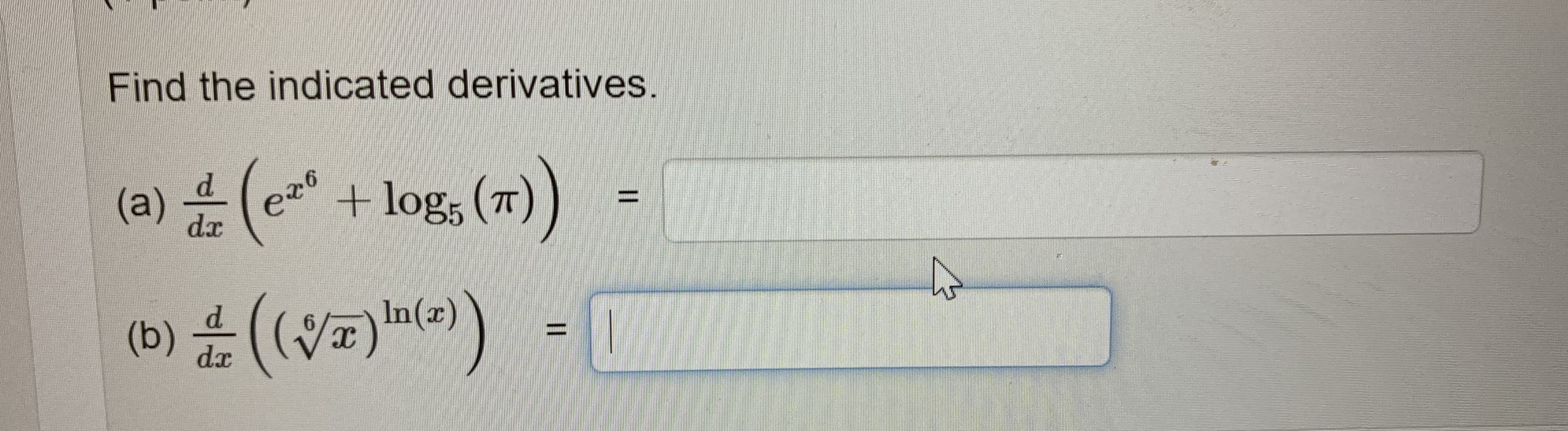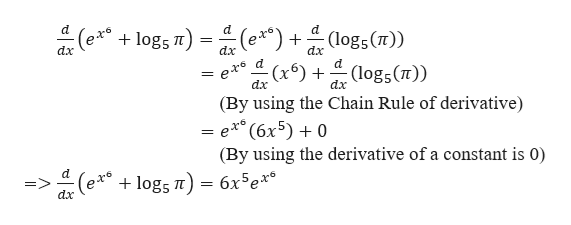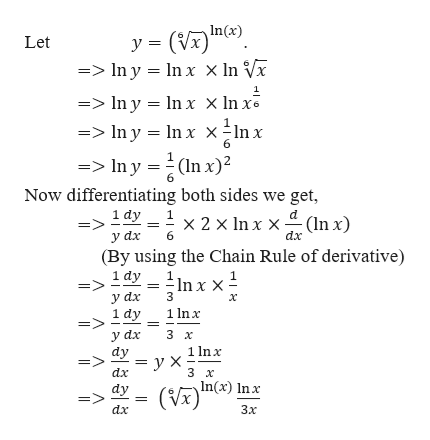# Find the indicated derivatives.(e*((v)e))+log5 (T(a)drIn(x)(b)dr

Question
31 views

As picture sho whelp_outlineImage TranscriptioncloseFind the indicated derivatives. (e* ((v)e)) +log5 (T (a) dr In(x) (b) dr fullscreen
check_circle

star
star
star
star
star
1 Rating
Step 1

We need to find the derivatives of the given expression.

Step 2

For (a),help_outlineImage Transcriptionclosed. d d :(logs(T)) logs T = dx dx dx d :(x6) : (logs(T)) dx (By using the Chain Rule of derivative exe (6x5)0 (By using the derivative of a constant is 0) (ets logs 6x5ex d. => dx fullscreen
Step 3

For (b),...help_outlineImage TranscriptioncloseIn(x) y (V =>Iny In x x In Vx Let 1 =>In y In x x In x => In y = ln x x -ln x =In y(ln x)2 Now differentiating both sides we get, 1 dy у аx (By using the Chain Rule of derivative) x 2 x ln x x (In x) dx 1 dy 3 y dx х 1 lnx 1 dy => y dx dy 3 х 1 nx ух 3 х dx dy In(x) In x dx Зх fullscreen

### Want to see the full answer?

See Solution

#### Want to see this answer and more?

Solutions are written by subject experts who are available 24/7. Questions are typically answered within 1 hour.*

See Solution
*Response times may vary by subject and question.
Tagged in

### Calculus